### Animated GIF GalleryRotating cube. [start | stop]

#### My First Rotation!

Once I finally thought I understood quaternions and wrote a Python class implementing them, this was my first effort at rotation.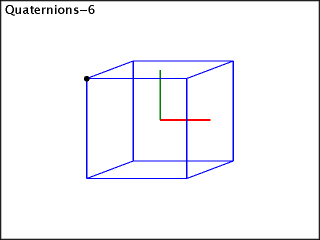Rotating cube. [start | stop]

#### Combining Rotations, Try #1

My first attempt to combine multiple rotation quaternions didn't turn out as planned. The idea was that one rotation (along the X axis) spun the cube on a horizontal axis, while a second rotation (along the Y axis) would rotate the spinning cube.

But the way the rotations interacted, the cube didn't maintain the horizontal orientation. This was because the rotation on the X axis was fixed. But after the Y axis rotated the cube, the X axis wasn't aligned with the cube anymore.

Notice how the red vector in the animation doesn't point at the same face of the cube.Rotating cube. [start | stop]

#### Combining Rotations, Try #2

The trick was using the second rotation (along the Y axis) to rotate the first quaterion, not the cube. The first quaternion spins the cube about its X axis — which remains fixed with the cube.

Notice how now the red vector — the rotated X-axis quaterion — always points to the same cube face dead on.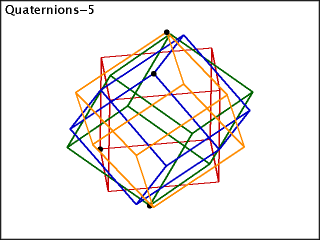Rotating cubes. [start | stop]

#### Multiple Rotating Boxes

This is just four separate boxes each being rotated on their own vector at their own rate. It looks almost hypnotic!

Pretty colors!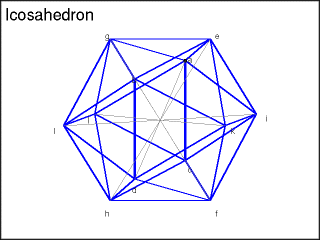. [start | stop]

#### Icosahedron

For my simple rotation experiments, I needed some shapes. I started with cubes, which are dead simple, but then thought I'd add the Platonic Solids (also pretty simple).

Here's my (rotating) icosahedron. I made it to help me figure out which points (the coordinates of which Wikipedia provided) connected to which points. The vectors (grey) from the origin to the points showed me the points, but I had no info on how to connect them, so it was a manual process.

Spinning the shape, made it easier to see the relationships of points and to verify my line-by-line connections were correct.. [start | stop]

#### Dodecahedron

Here's my (rotating) dodecahedron. Same deal as above.. [start | stop]

#### The Rotating Tesseract

The point of all of this is my fascination with tesseracts. The ultimate goal is to fully understand them.

In particular to understand exactly what's happening in the canonical "rotating tesseract" one frequently sees. (Such as the one here.)

The irony is that quaternions aren't involved in this part at all (but it was fun figuring them out). It's not even entirely clear to me that trigonomic functions are part of this — as they would be for ordinary rotations.

In this example, the transformation is done with a sin function, but it only serves to provide a [0, 1) range of values. It's possible a simple linear transform works just fine. (It seems like one aspect should be using sin while another uses cos, but so far I'm not seeing a need.)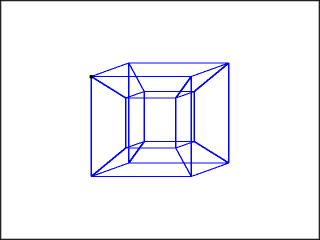. [start | stop]

#### Oops! (#1)

Things don't always go as planned. This was supposed to be like the one above, but rotating on the Y-axis, too.

It didn't quite.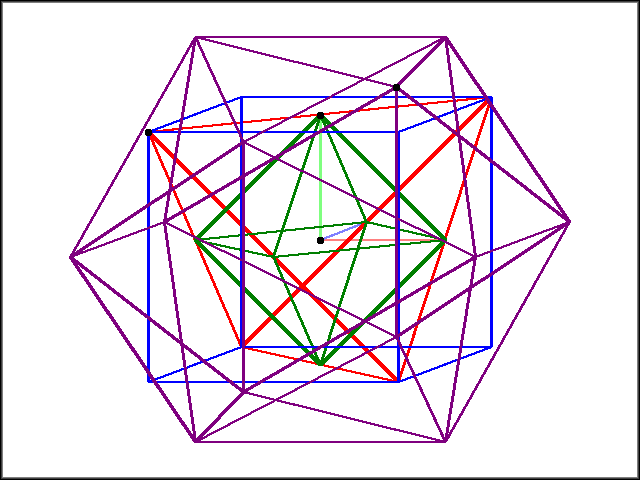. [start | stop]

#### (more to come)

Watch this space…